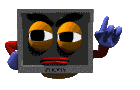Formatting Output

#include <iostream.h>
#include <iomanip.h>

Formatting output is important in the development of output screens which can be easily read and understood.  C++ offers the programmer several input/output manipulators.  Two of these I/O manipulators are setw( ) and setprecision( )The setw( ) manipulator sets the width of the field assigned for the output.  It takes the size of the field (in number of characters) as a parameter.

For example, the code:    cout << setw(6) <<"R";
generates the following output on the screen (each underscore represents a blank space)

_ _ _ _ _R

The setw( ) manipulator does not stick from one cout statement to the next.  For example, if you want to right-justify three numbers within an 8-space field, you will need to repeat setw( ) for each value:
cout << setw(8) << 22 << "\n";
cout << setw(8) << 4444 << "\n";
cout << setw(8) << 666666 << endl;

The output will be (each underscore represents a blank space)
_ _ _ _ _ _ 2 2
_ _ _ _ 4 4 4 4
_ _ 6 6 6 6 6 6The setprecision( ) manipulator sets the total number of digits to be displayed when floating point numbers are printed.

For example, the code:    cout << setprecision(5) << 123.456;
will print the following output to the screen (notice the rounding):

123.46

The setprecision( ) manipulator can also be used to set the number of decimal places to be displayed.   In order for setprecision( ) to accomplish this task, you will have to set an ios flag.  The flag is set with the following statement:

cout.setf(ios::fixed);

Once the flag has been set, the number you pass to setprecision( ) is the number of decimal places you want displayed.  The following code:

cout.setf(ios::fixed);
cout << setprecision(5) << 12.345678;

generates the following output on the screen (notice no rounding):

12.34567Additional IOS flags: In the statement:  cout.setf(ios::fixed); "fixed" is referred to as a format option.

Other possible format options:

 left left-justify the output right right-justify the output showpoint display decimal point and trailing zeros for all floating point numbers, even if the decimal places are not needed. uppercase display the "e" in E-notation as "E" rather than "e" showpos display a leading plus sign before positive values scientific display floating point numbers in scientific ("E") notation fixed display floating point numbers in normal notation - no trailing zeroes and no scientific notation

**Note: You can remove these options by replacing setf with unsetf.

Displaying Amounts of Money:
To get 5.8 to display as 5.80, the following lines of code are needed"

//display money

cout.setf(ios::fixed);
cout.setf(ios::showpoint);
cout << setprecision(2);
c
out << 5.8;

 All subsequent couts retain the precision set with the last setprecision( ). Setprecision( ) is "sticky."  Whatever precision you set, sticks with the cout device until such time as you change it with an additional setprecision( ) later in the program.